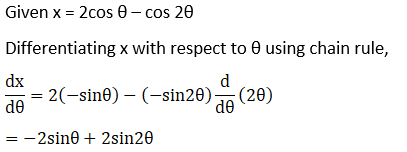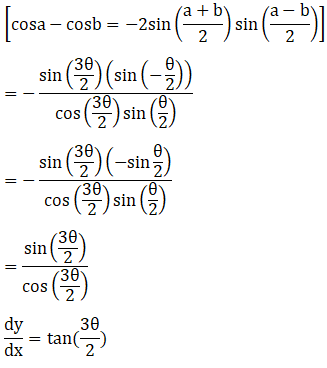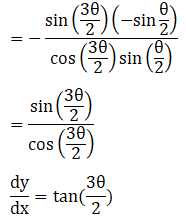# RD Sharma Solutions For Class 12 Maths Exercise 11.7 Chapter 11 Differentiation

RD Sharma Solutions for Class 12 Maths Exercise 11.7 Chapter 11 differentiation is provided here. The RD Sharma book comprises a vast number of well-graded exercises and solved examples which improves problem-solving skills among students. This exercise explains the differentiation of parametric functions. Parametric functions are functions of a number of coordinates, where each coordinate is expressed as another function of some parameter.

Students can effectively start practising RD Sharma Solutions for Class 12, which will build a strong precision in the subject and in turn, will result in better performance in board exams. To know more about these concepts they are required to go through RD Sharma Solutions for Class 12 Maths Chapter 11 Exercise 11.7 from the below provided links. These solutions are designed by a team of experts at BYJU’S to serve the students.

## RD Sharma Solutions For Class 12 Differentiation Exercise 11.7:-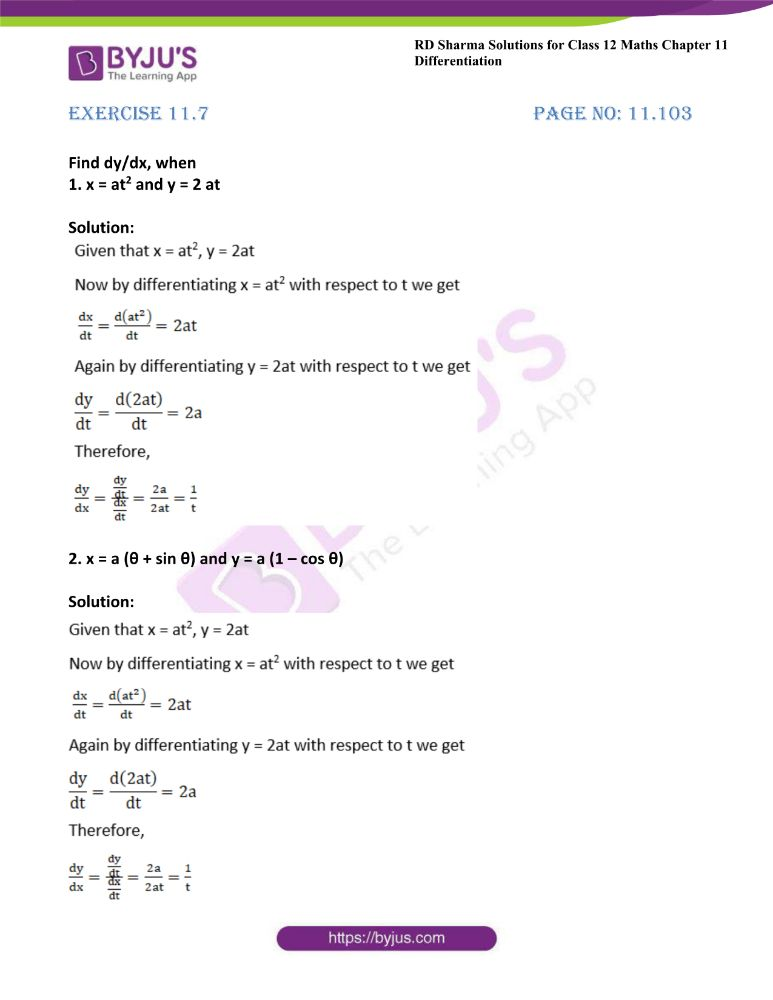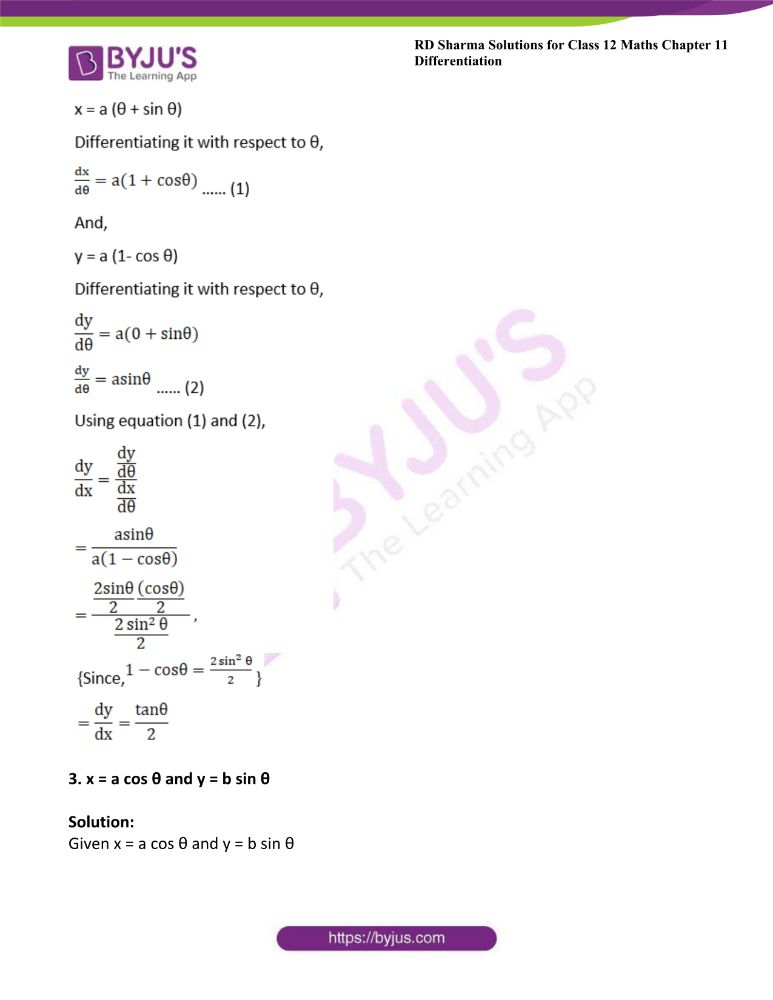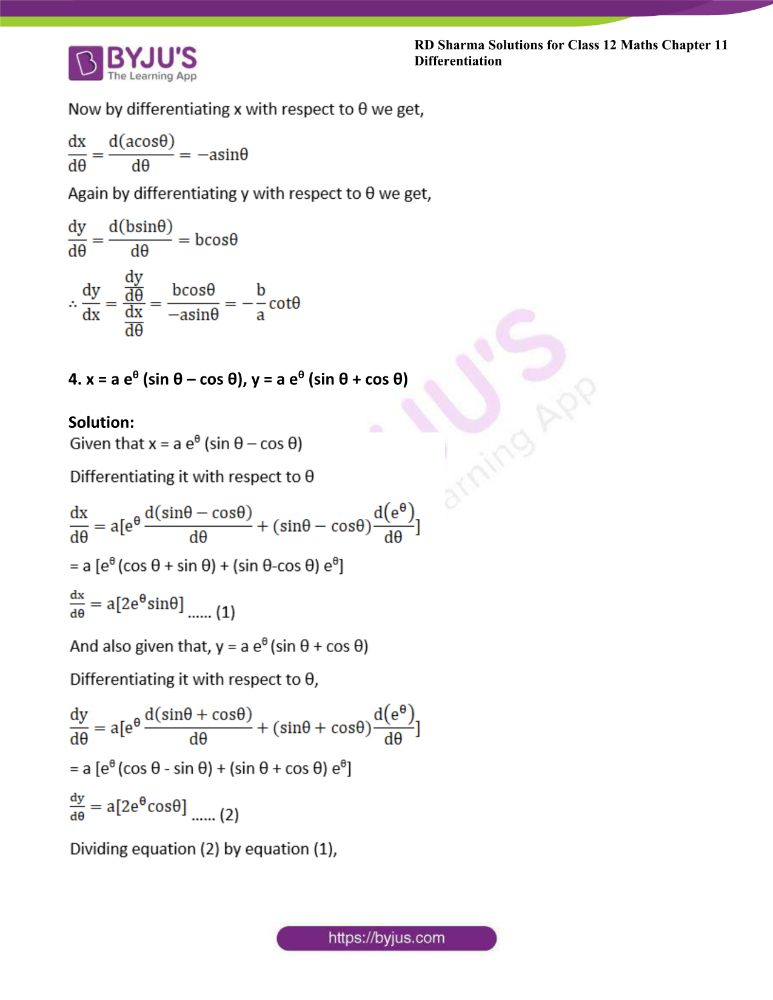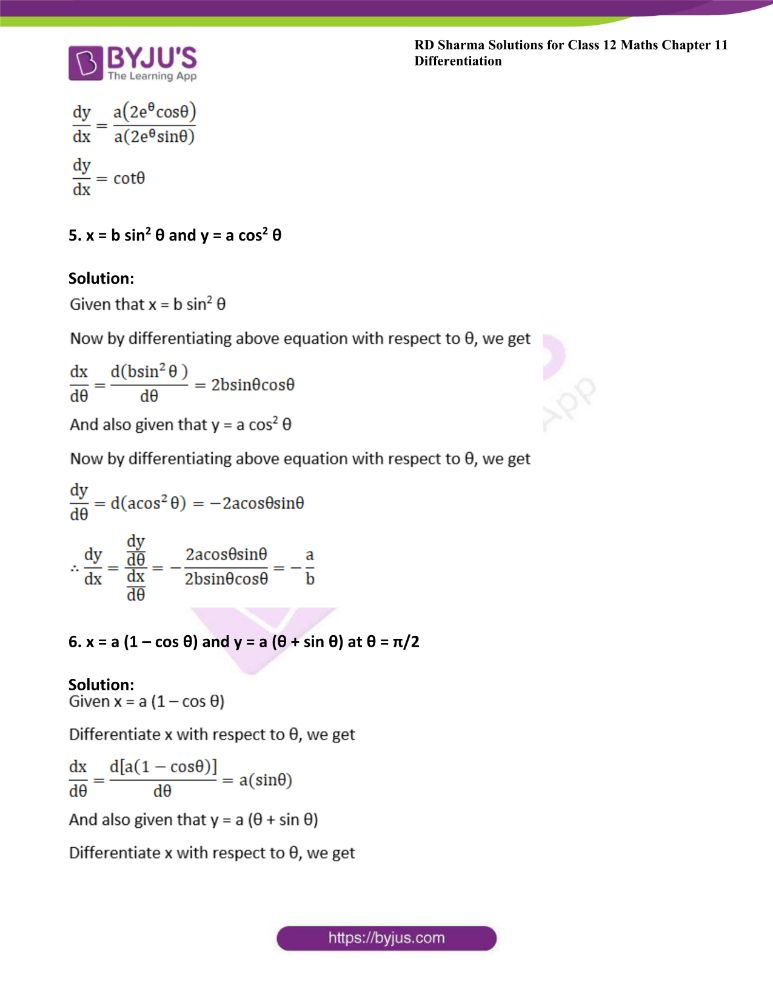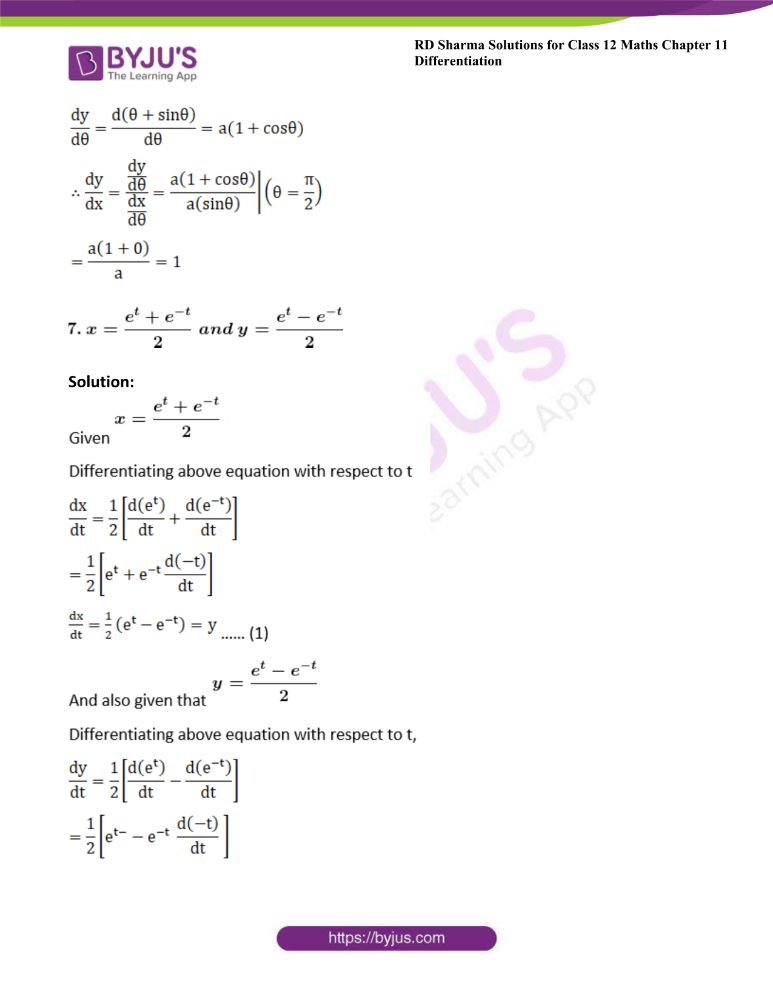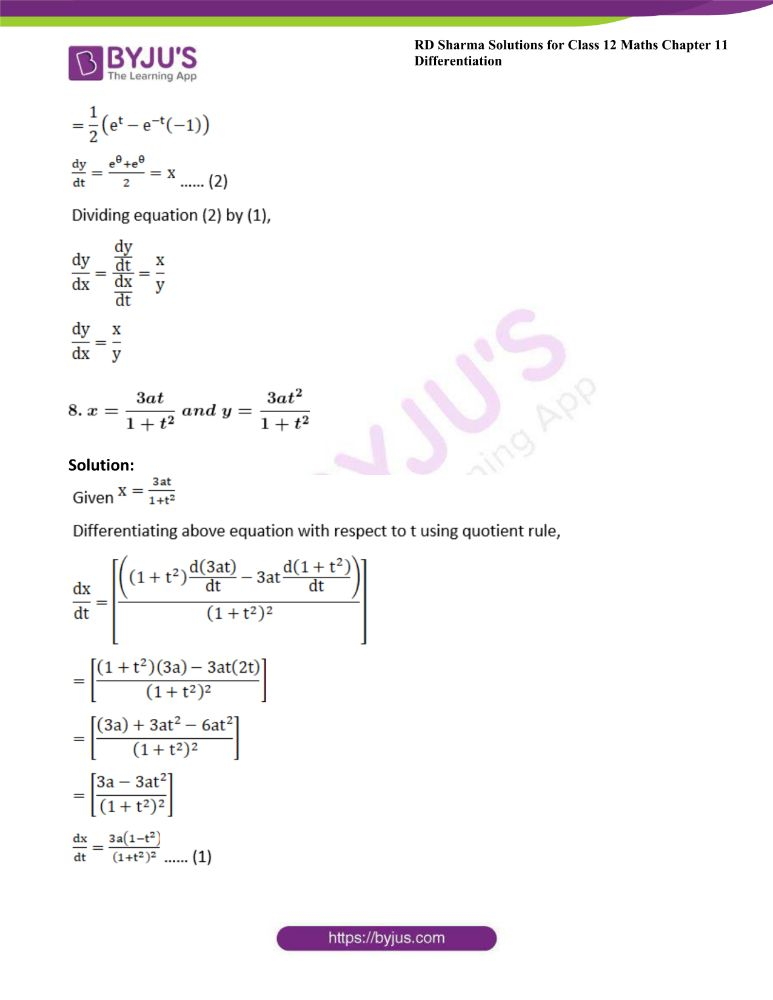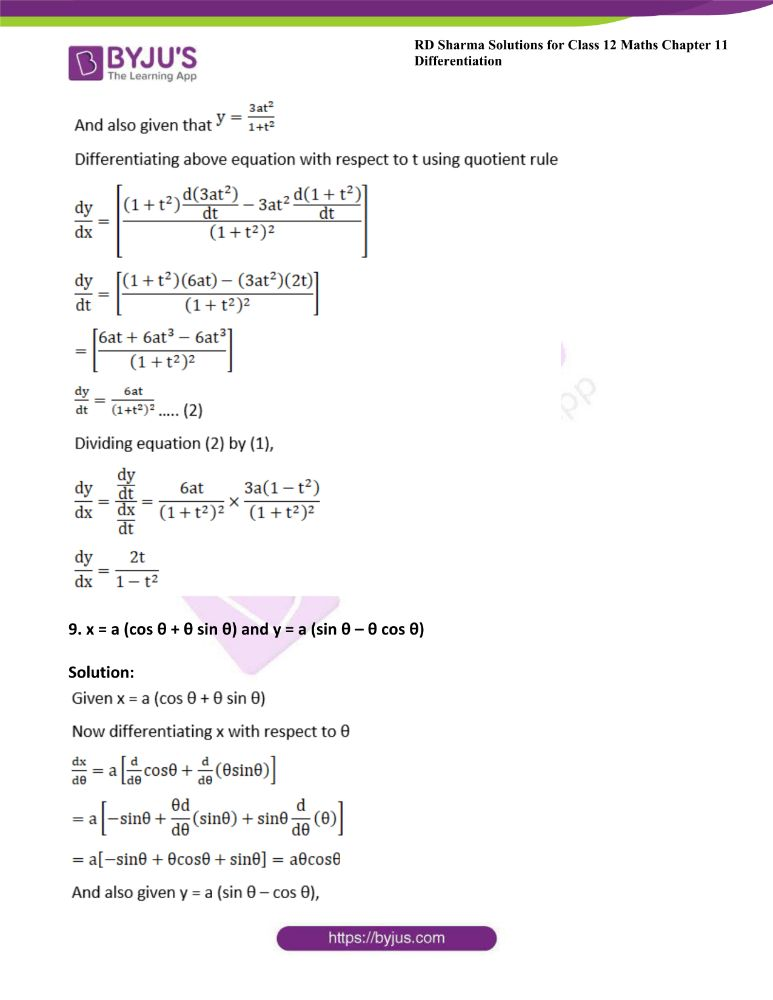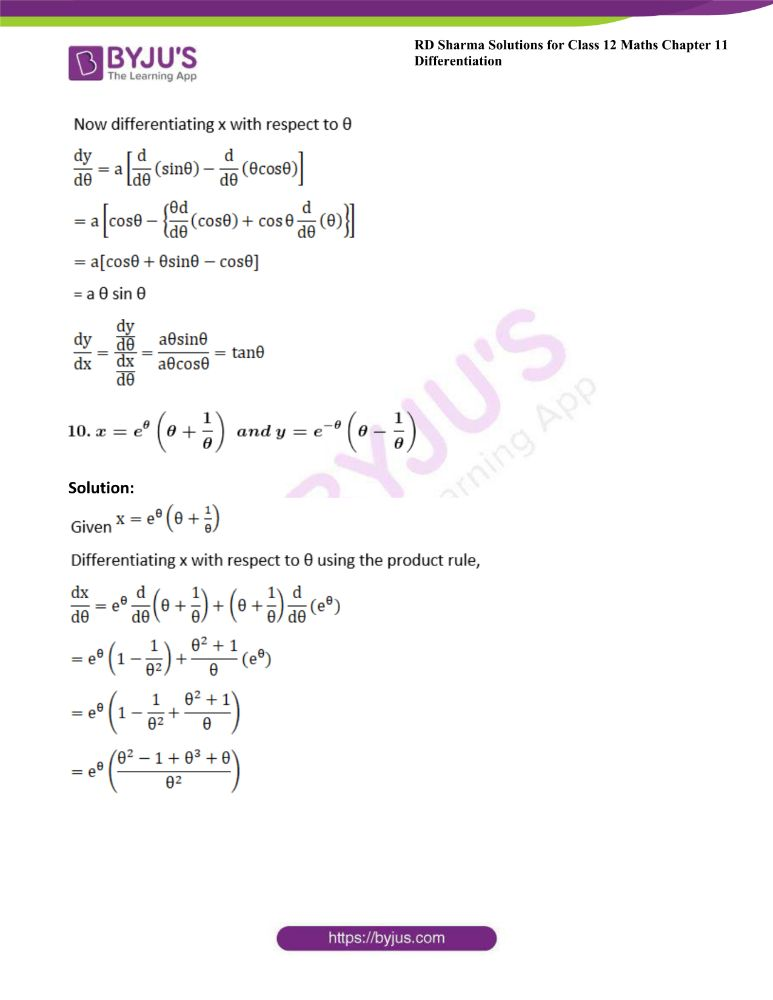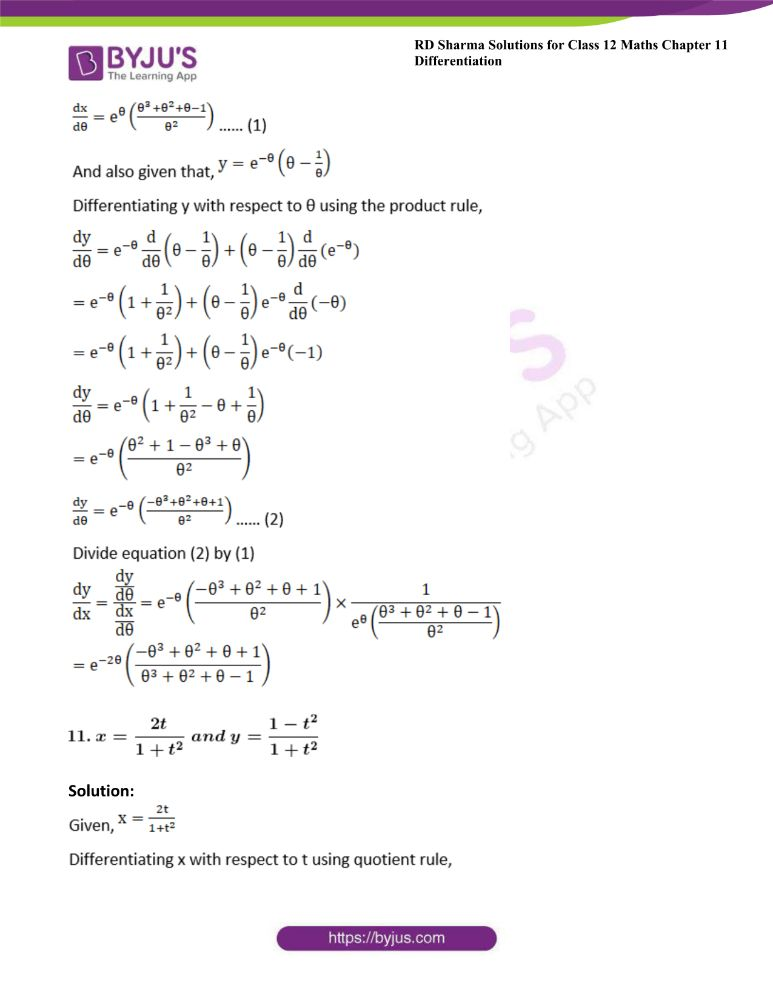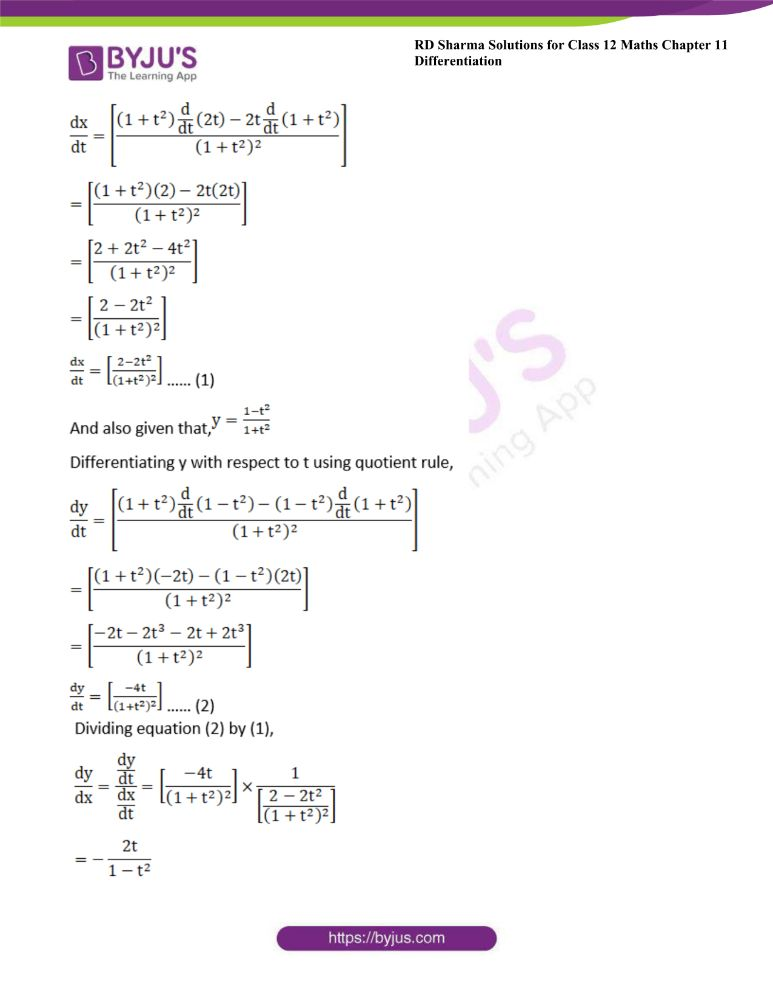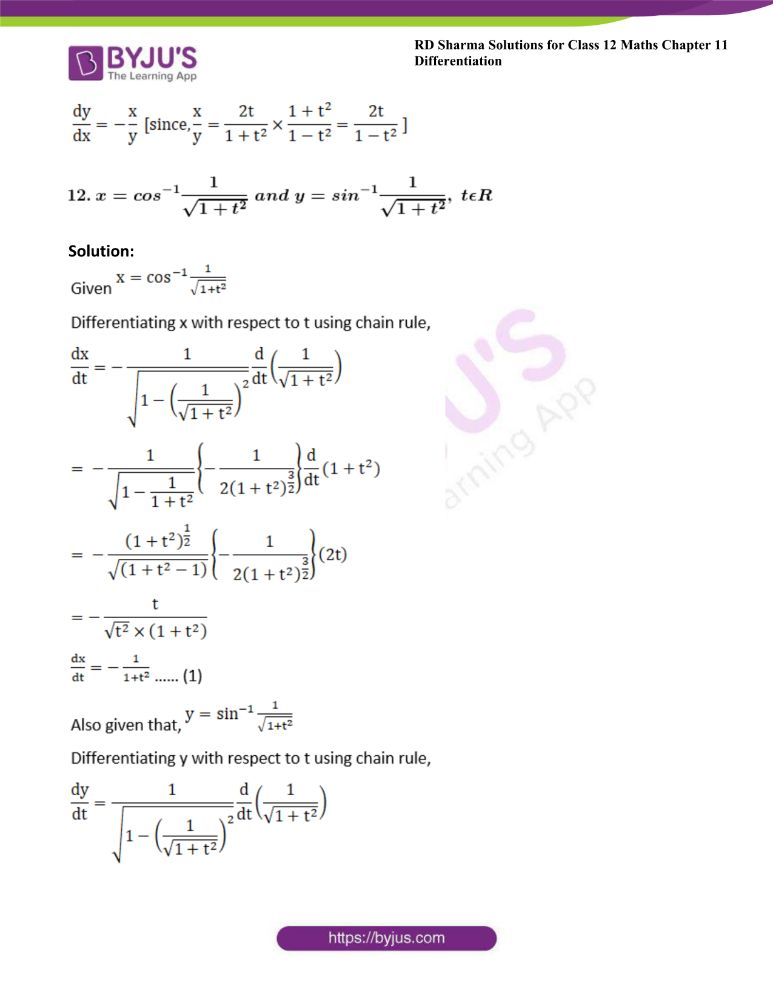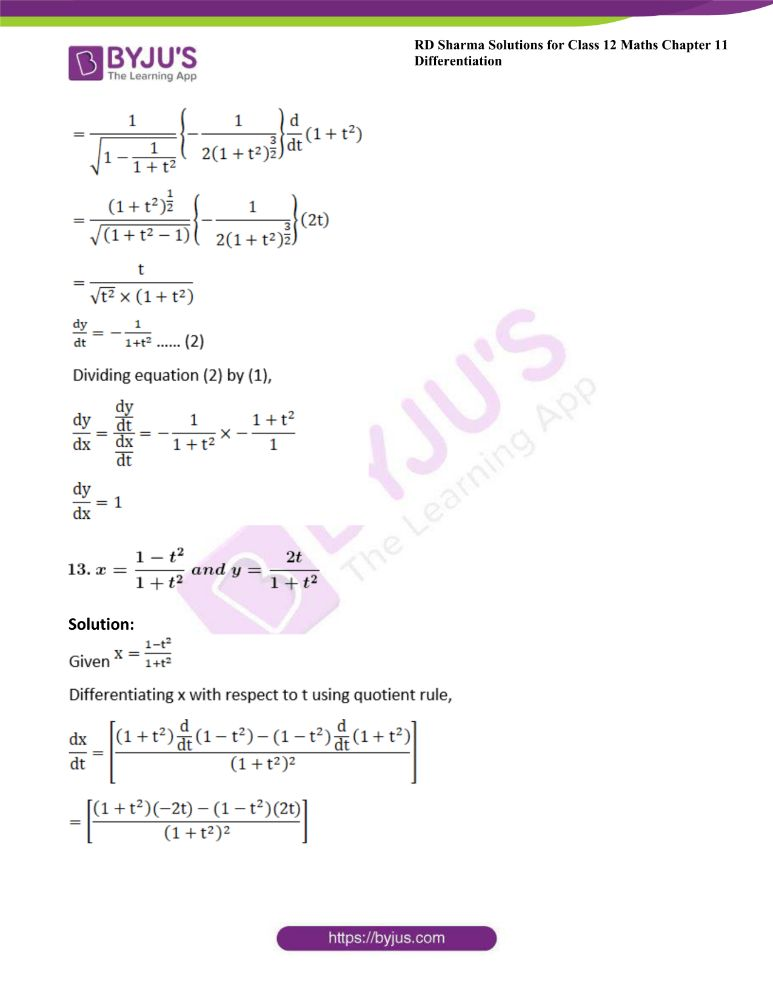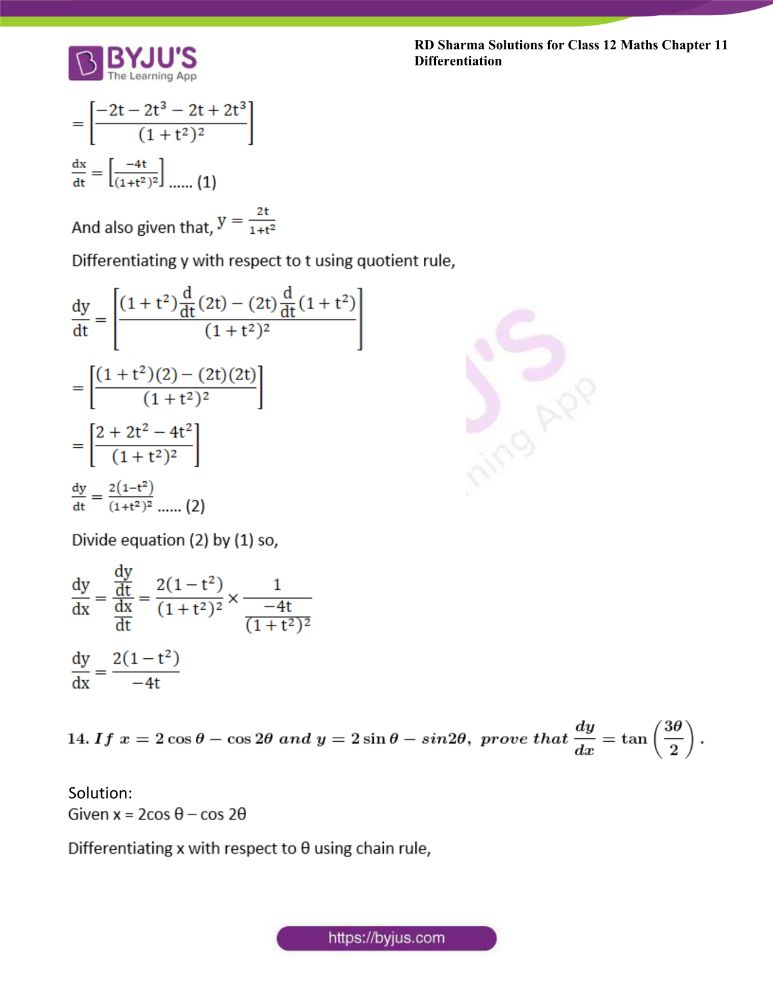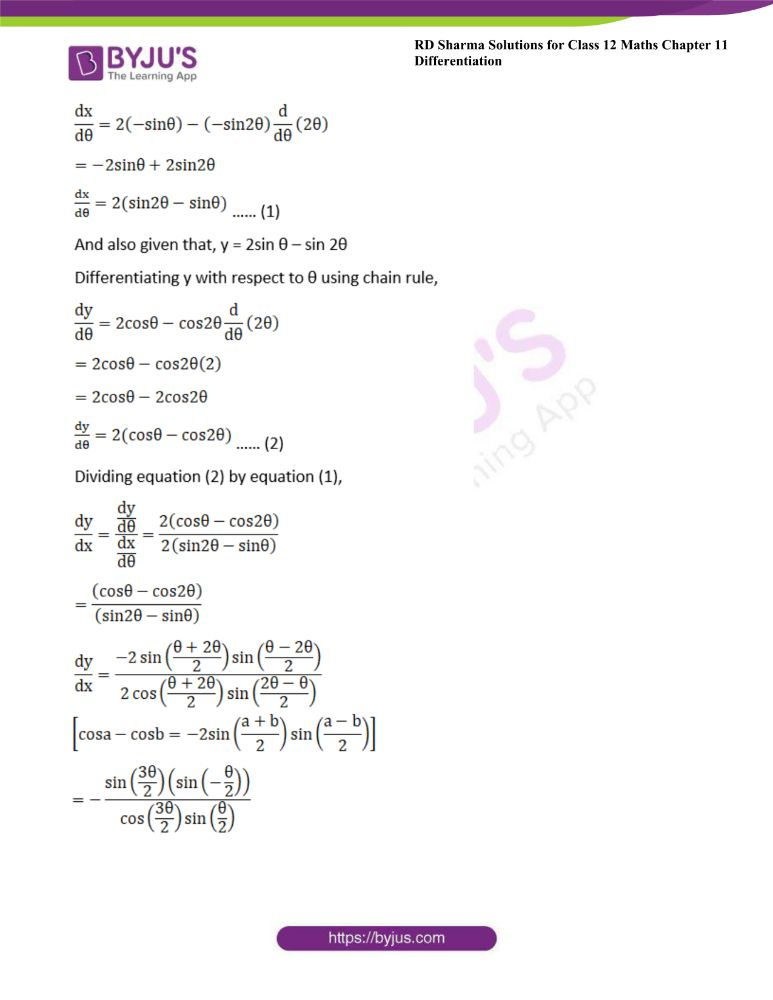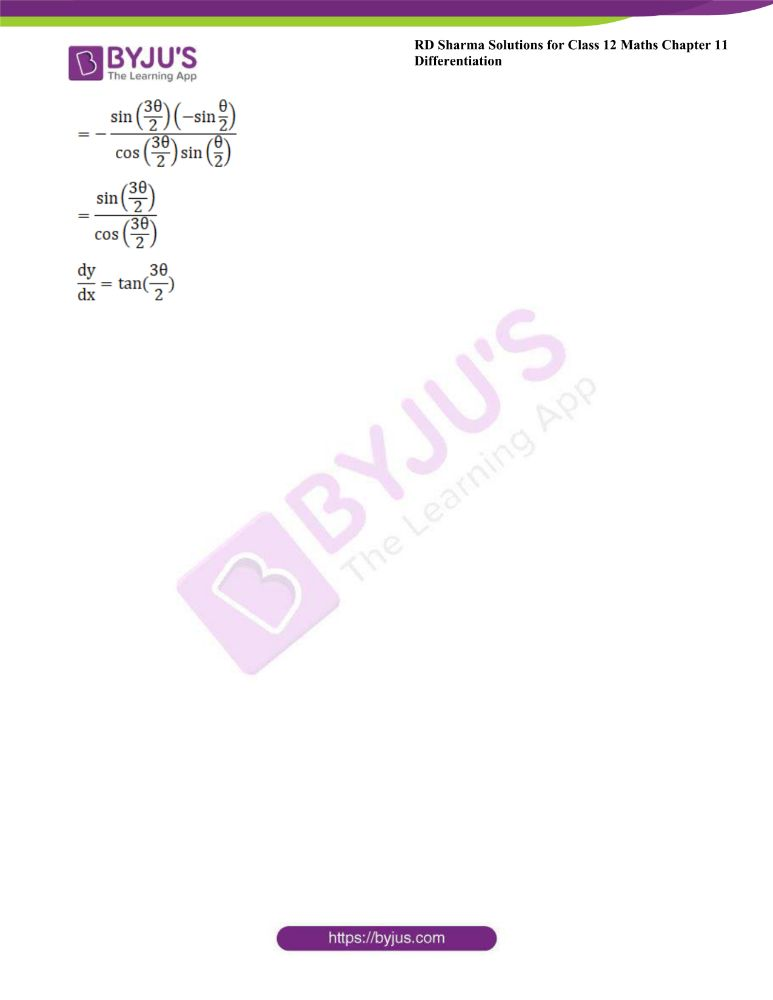### Access other exercises of RD Sharma Solutions For Class 12 Chapter 11 – Differentiation

Exercise 11.1 Solutions

Exercise 11.2 Solutions

Exercise 11.3 Solutions

Exercise 11.4 Solutions

Exercise 11.5 Solutions

Exercise 11.6 Solutions

Exercise 11.8 Solutions

### Access answers to Maths RD Sharma Solutions For Class 12 Chapter 11 – Differentiation Exercise 11.7

Exercise 11.7 Page No: 11.103

Find dy/dx, when

1. x = at2 and y = 2 at

Solution: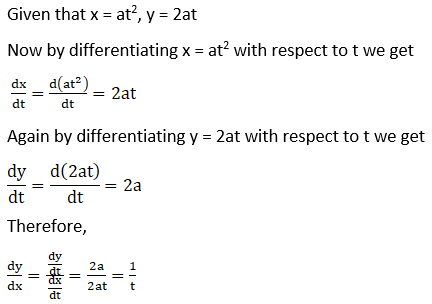2. x = a (θ + sin θ) and y = a (1 – cos θ)

Solution: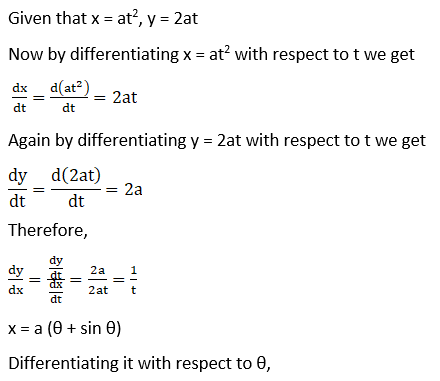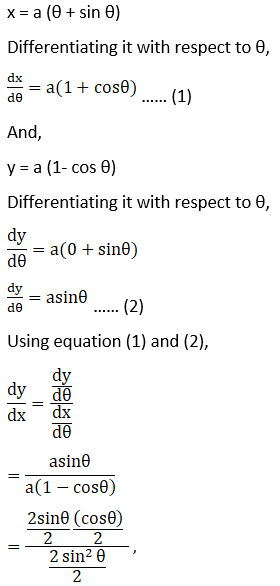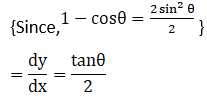3. x = a cos θ and y = b sin θ

Solution:

Given x = a cos θ and y = b sin θ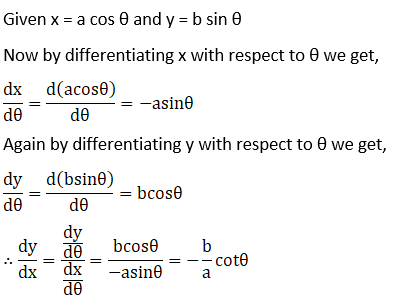4. x = a eθ (sin θ – cos θ), y = a eθ (sin θ + cos θ)

Solution: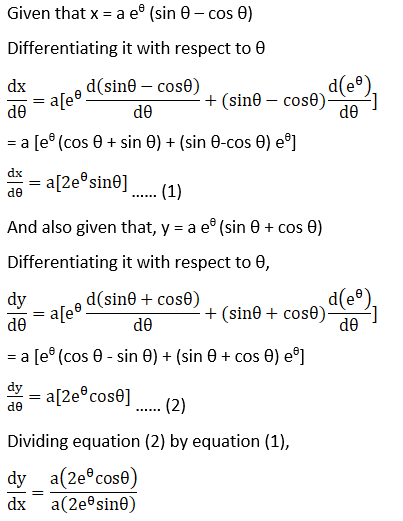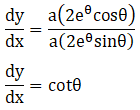5. x = b sin2 θ and y = a cos2 θ

Solution: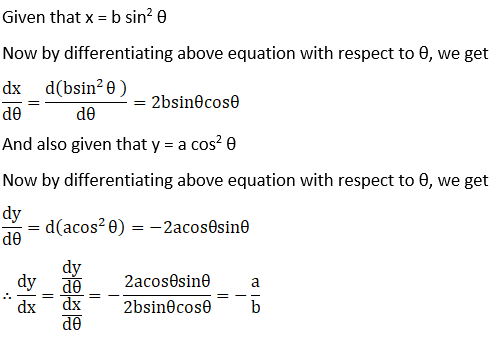6. x = a (1 – cos θ) and y = a (θ + sin θ) at θ = π/2

Solution: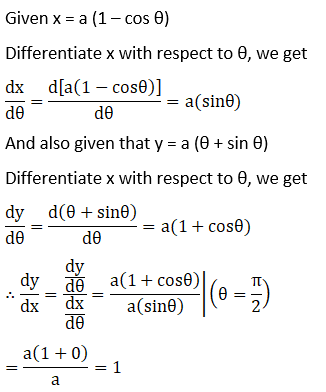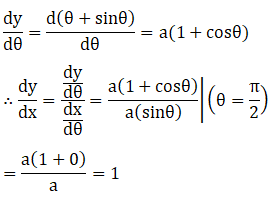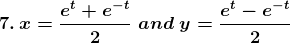Solution: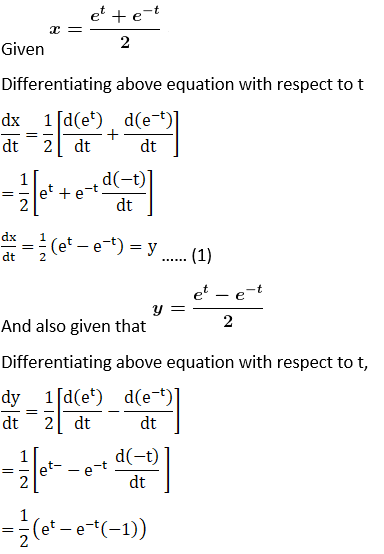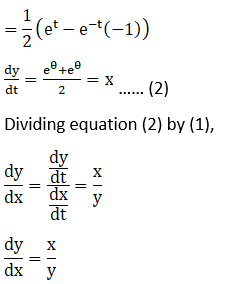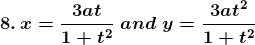Solution: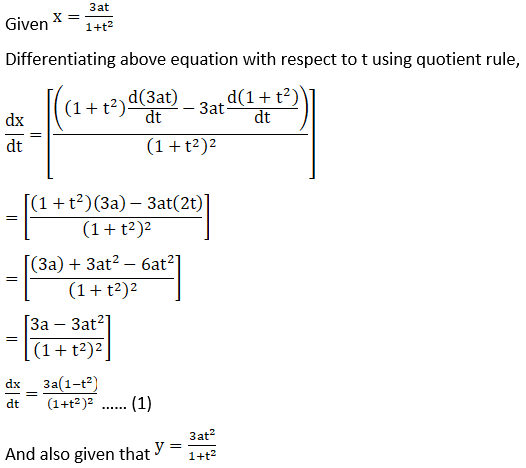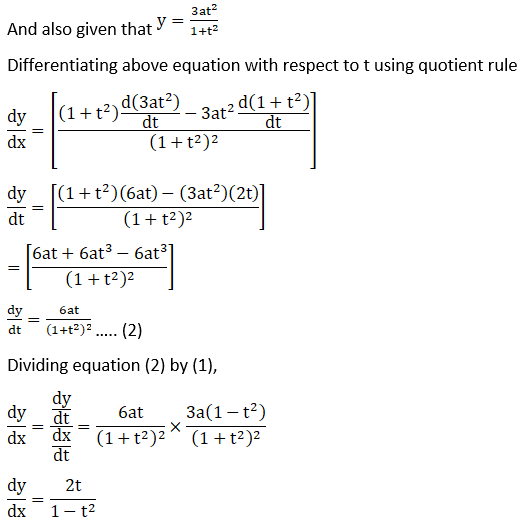9. x = a (cos θ + θ sin θ) and y = a (sin θ – θ cos θ)

Solution: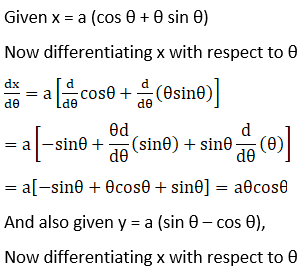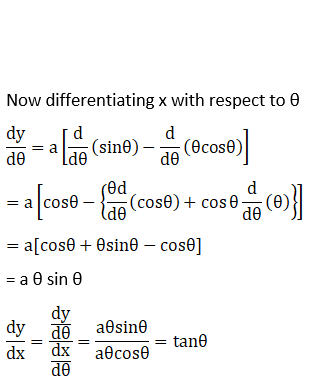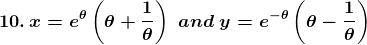Solution: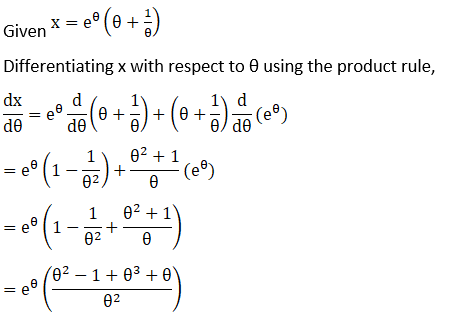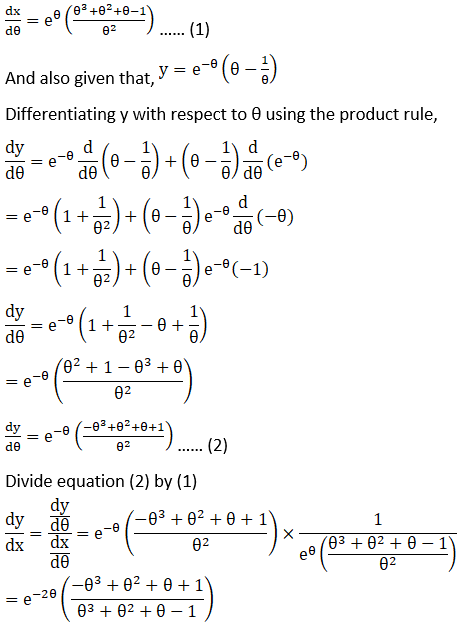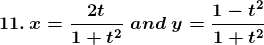Solution: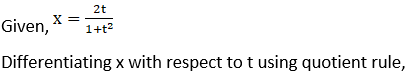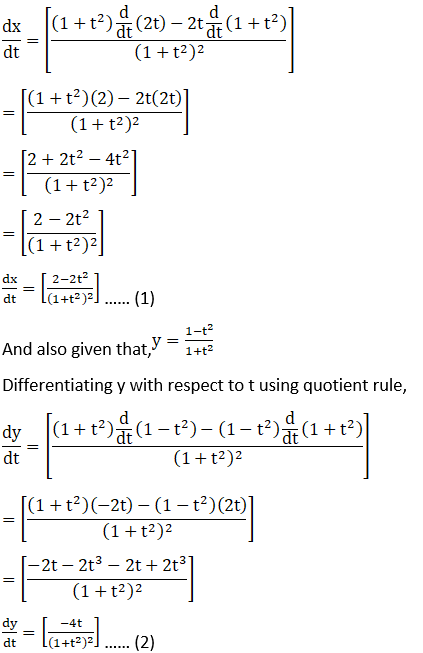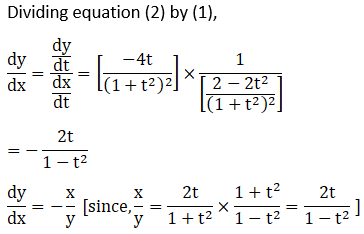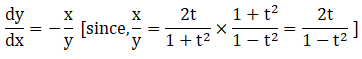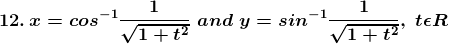Solution: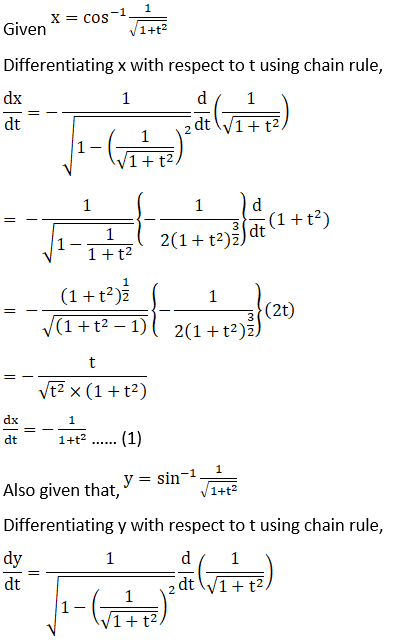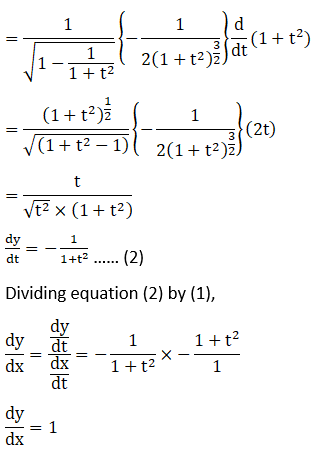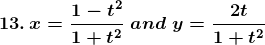Solution: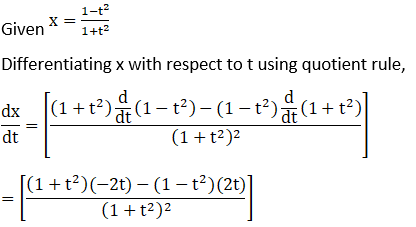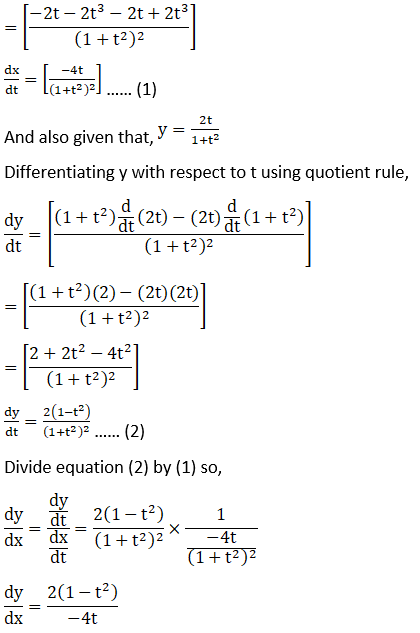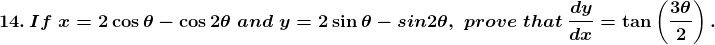Solution: